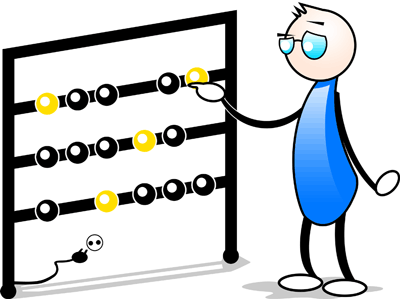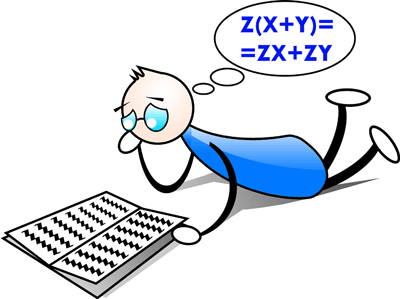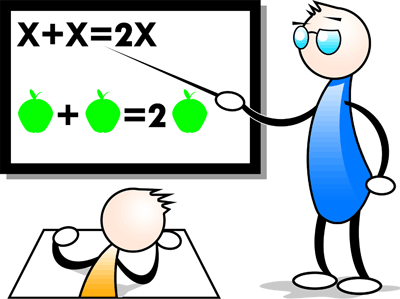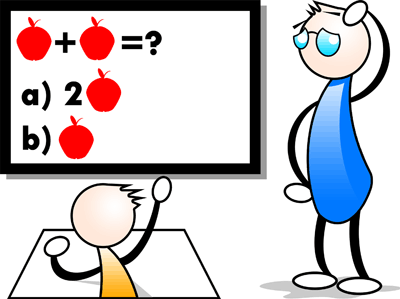Math Calculators, Lessons and Formulas

It is time to solve your math problem

mathportal.org

# Free calculators, formulas & lessons